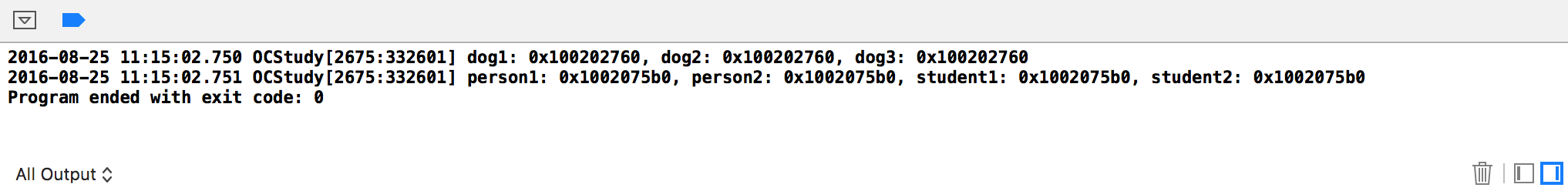# 1、单例类的使用# 2、单例类的实现方式

• 创建一个单例类；

• 提供了一个类方法sharedInstance，通过该方法就能够获取到该单例类的对象；

• 提供了copy方法，调用copy方法时，也是返回已经存在的单例类对象；

（注意：由于ARC已经成为主流，所以以下的单例类创建方法只考虑ARC）

• .h文件
``````#import <Foundation/Foundation.h>
@interface Singleton : NSObject <NSCopying>
/**
*  获取单例类
*
*  @return 单例类对象
*/
+(instancetype) sharedInstance;
@end
``````
• .m文件
``````#import "Singleton.h"
@implementation Singleton

static id _instance;

+ (instancetype)sharedInstance
{
static dispatch_once_t onceToken;
dispatch_once(&onceToken, ^{
_instance = [[self alloc] init];
});
return _instance;
}

+ (id)allocWithZone:(struct _NSZone *)zone
{
static dispatch_once_t onceToken;
dispatch_once(&onceToken, ^{
_instance = [super allocWithZone:zone];
});
return _instance;
}

- (id)copyWithZone:(NSZone *)zone
{
return _instance;
}
@end
``````

# 3、示例代码

``````#import <Foundation/Foundation.h>
#import "MYDog.h"
#import "MYPerson.h"
#import "MYStudent.h"
int main(int argc, const char * argv[]) {
@autoreleasepool {
MYDog *dog1 = [MYDog sharedInstance];
MYDog *dog2 = [[MYDog alloc] init];
MYDog *dog3 = [dog1 copy];
NSLog(@"dog1: %p, dog2: %p, dog3: %p",dog1,dog2,dog3);
MYPerson *person1 = [[MYPerson alloc] init];
MYPerson *person2 = [MYPerson sharedInstance];
MYStudent *student1 = [[MYStudent alloc] init];
MYStudent *student2 = [MYStudent sharedInstance];
NSLog(@"person1: %p, person2: %p, student1: %p, student2: %p",person1,person2,student1,student2);
}
return 0;
}
``````https://github.com/99ios/15.2